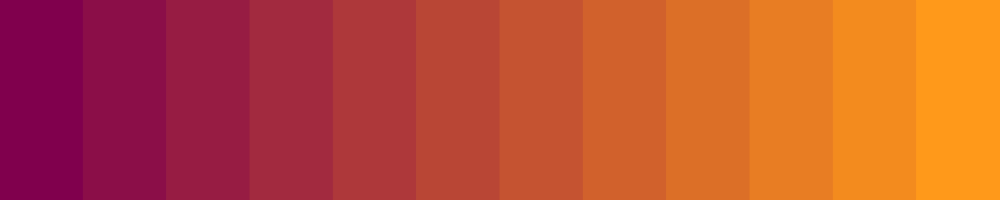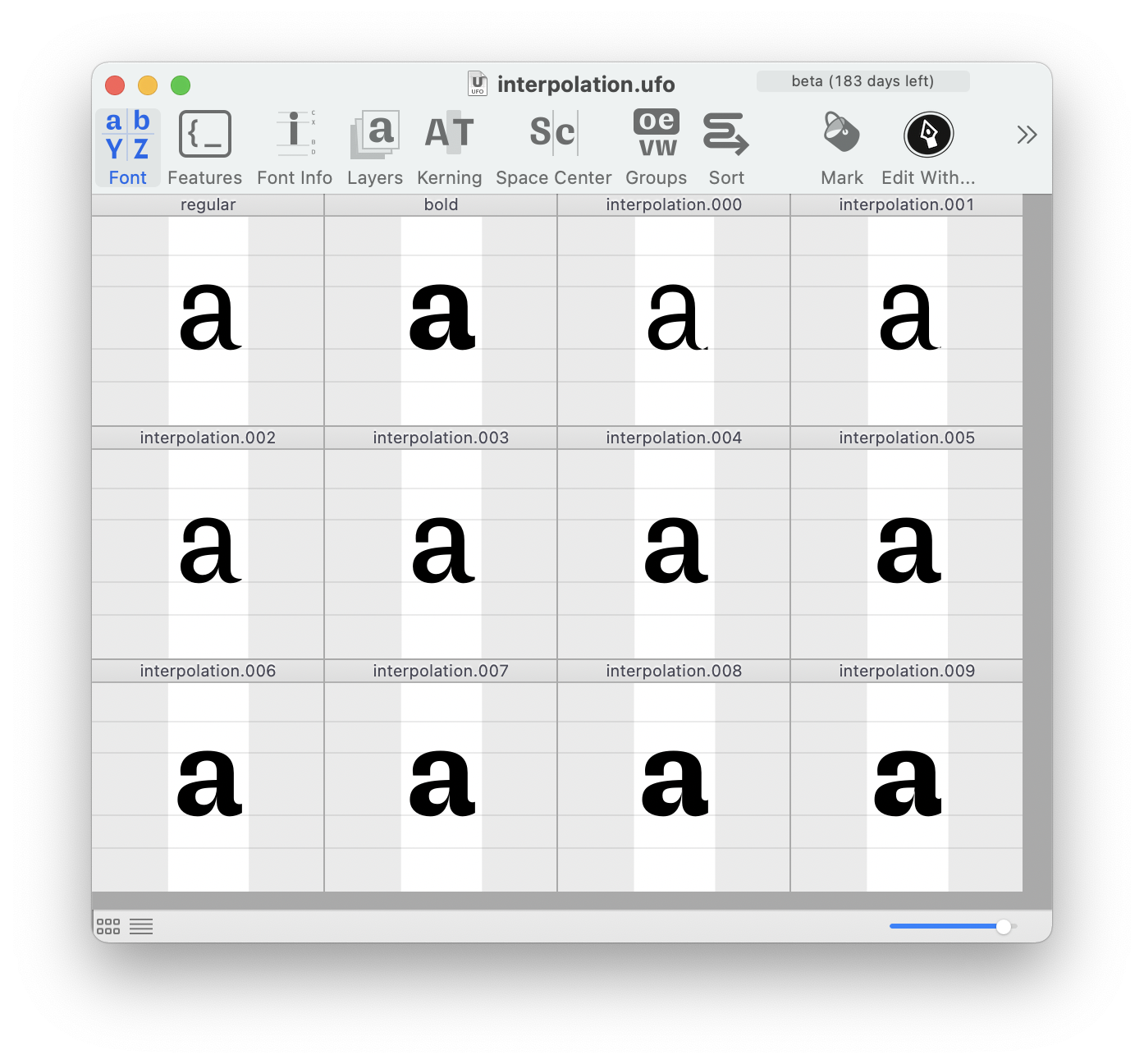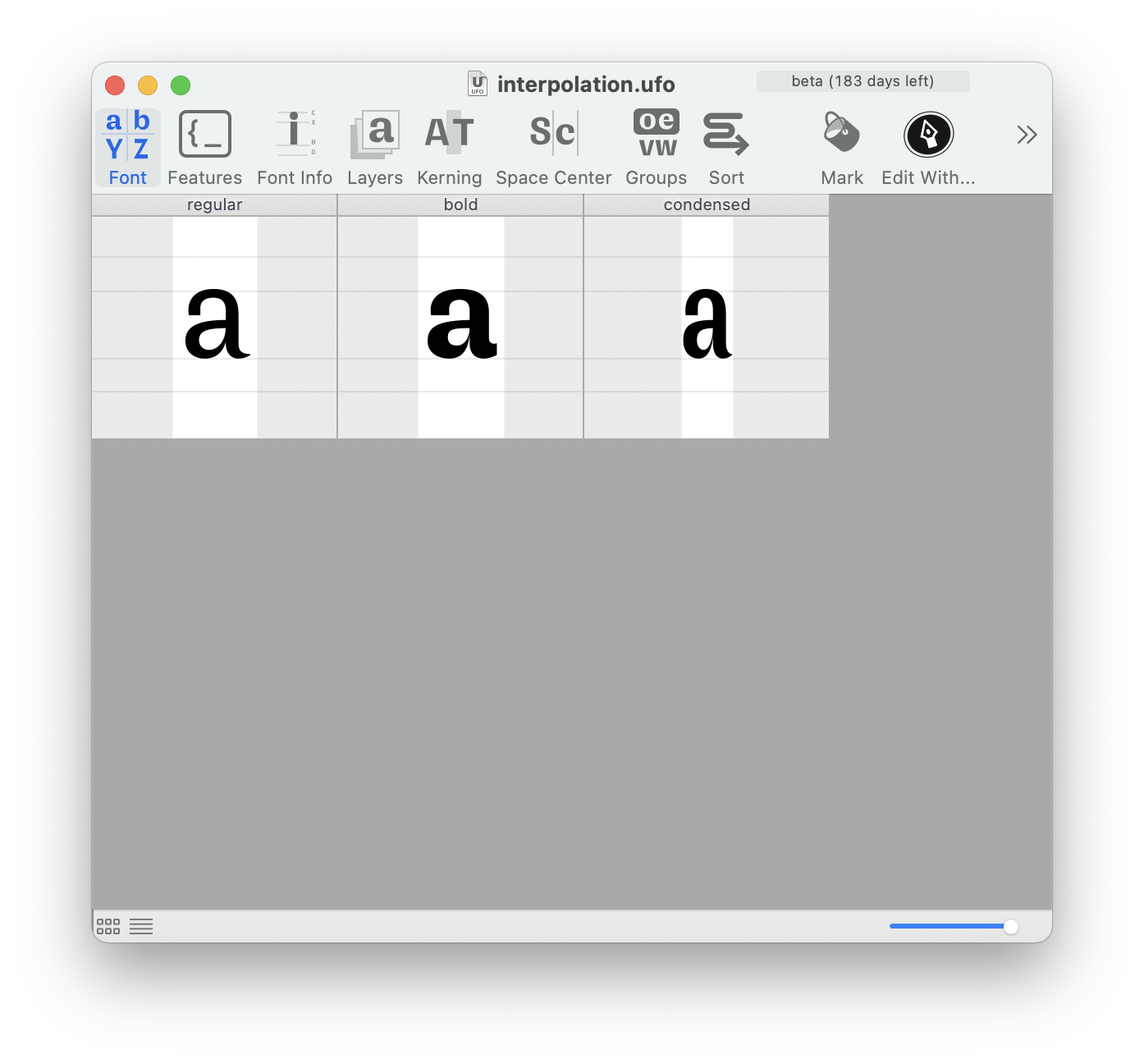## Interpolating colors

This example shows how to interpolate two RGB colors by interpolating each R, G, B color value separately.``````size(1000, 200)

# define two rgb colors
color1 = 0.5, 0.0, 0.3
color2 = 1.0, 0.6, 0.1

# total number of steps
steps = 12

# initial position
x, y = 0, 0

# calculate size for steps
w = width() / steps
h = height()

# iterate over the total amount of steps
for i in range(steps):

# calculate interpolation factor for this step
factor = i * 1.0 / (steps - 1)

# interpolate each rgb channel separately
r = color1 + factor * (color2 - color1)
g = color1 + factor * (color2 - color1)
b = color1 + factor * (color2 - color1)

# draw a rectangle with the interpolated color
fill(r, g, b)
stroke(r, g, b)
rect(x, y, w, h)

# increase x-position for the next step
x += w
``````

## Interpolating position and size

The next example shows the same approach used to interpolate position and size.``````# define position and size for two rectangles
x1, y1 = 98, 88
w1, h1 = 480, 492

x2, y2 = 912, 794
w2, h2 = 20, 126

# total amount of steps
steps = 22

# define blend mode and color
blendMode('multiply')
fill(0, 0.3, 1, 0.2)

# iterate over the total amount of steps
for i in range(steps):

# calculate interpolation factor for this step
factor = i * 1.0 / (steps - 1)

# interpolate each rectangle attribute separately
x = x1 + factor * (x2 - x1)
y = y1 + factor * (y2 - y1)
w = w1 + factor * (w2 - w1)
h = h1 + factor * (h2 - h1)

# draw a rectangle with the calculated variables
rect(x, y, w, h)
``````

## Checking interpolation compatibility

Before interpolating two glyphs, we need to make sure that they are compatible. We can do that with code using a glyph’s `isCompatible` method. This function returns two values:

• The first is a boolean indicating if the two glyphs are compatible.
• If the first value is `False`, the second will contain a report of the problems.
``````f = CurrentFont()
g = f['O']
print(g.isCompatible(f['o']))
``````
``````(True, '')
``````
``````print(g.isCompatible(f['n']))
``````
``````(False, '[Fatal] Contour 0 contains a different number of segments.\n[Fatal] Contour 1 contains a different number of segments.\n[Warning] The glyphs do not contain components with exactly the same base glyphs.')
``````

## Interpolating glyphs in the same font

The script below shows how to generate a number of interpolation and extrapolation steps between two selected glyphs in the current font.``````# settings
interpolationSteps = 5
extrapolateSteps = 2

# get the currentFont
f = CurrentFont()

if f is None:
# no font open
print("Oeps! There is not font open.")

else:
# get the selection
selection = f.selectedGlyphNames

# check if the selection contains only two glyphs
if len(selection) != 2:
print("Incompatible selection: two compatible glyphs are required.")

else:
# get the master glyphs
source1 = f[selection]
source2 = f[selection]

# check if they are compatible
if not source1.isCompatible(source2):
# the glyphs are not compatible
print("Incompatible masters: Glyph %s and %s are not compatible." % (source1.name, source2.name))

else:
# loop over the amount of required interpolations
nameSteps = 0
for i in range(-extrapolateSteps, interpolationSteps + extrapolateSteps + 1, 1):
# create a new name
name = "interpolation.%03i" % nameSteps
nameSteps += 1
# create the glyph if does not exist
dest = f.newGlyph(name)
# get the interpolation factor (a value between 0.0 and 1.0)
factor = i / float(interpolationSteps)
# interpolate between the two masters with the factor
dest.interpolate(factor, source1, source2)

# done!
f.changed()
``````

## Interpolating between two sources

This example shows how to interpolate glyphs from two source fonts into a third font.

The script expects 3 fonts open:

1. the current font, where the glyphs will be interpolated
2. one source font named “Regular”
3. another source font named “Bold”
``````# the font where the interpolated glyphs will be stored
f = CurrentFont()

# the two master fonts
f1 = AllFonts().getFontsByStyleName('Regular')
f2 = AllFonts().getFontsByStyleName('Bold')

# the interpolation factor
factor = 0.4

# a list of glyph names to be interpolated
glyphNames = ['A', 'B', 'C', 'a', 'b', 'c']

# iterate over the glyph names
for glyphName in glyphNames:

# if this glyph is not available in one of the masters, skip it
if glyphName not in f1:
print(f'{glyphName} not in {f1}, skipping…')
continue
if glyphName not in f2:
print(f'{glyphName} not in {f2}, skipping…')
continue

# if the glyph does not exist in the destination font, create it
if glyphName not in f:
f.newGlyph(glyphName)

# interpolate glyph
print(f'interpolating {glyphName}…')
f[glyphName].interpolate(factor, f1[glyphName], f2[glyphName])

``````

## Interpolating fonts

Generate a new font by interpolating two source fonts.

``````# get fonts
font1 = OpenFont('path/to/font1.ufo')
font2 = OpenFont('path/to/font2.ufo')

# define interpolation factor
factor = 0.5

# make destination font
font3 = NewFont()

# this interpolates the glyphs
font3.interpolate(factor, font1, font2)

# this interpolates the kerning
# comment this line out of you're just testing
font3.kerning.interpolate(factor, font1.kerning, font2.kerning)

# done!
font3.save('path/to/font3.ufo')

``````

## Batch interpolating fonts

The following example generates a set of instances by interpolating two source fonts.

The names and interpolation values of each instance are defined in a list of tuples.

``````import os
from vanilla.dialogs import getFolder

# get masters and destination folder
font1 = OpenFont('path/to/font1.ufo')
font2 = OpenFont('path/to/font2.ufo')
folder = getFolder("Select a folder to save the interpolations")

# instance names and interpolation factors
instances = [
("Light", 0.25),
("Regular", 0.5),
("Bold", 0.75),
]

# generate instances
print('generating instances...\n')
for instance in instances:
styleName, factor = instance
print(f"\tgenerating {styleName} ({factor})...")

# make a new font
destFont = NewFont()

# interpolate the glyphs
destFont.interpolate(factor, font1, font2)

# set font name
destFont.info.familyName = "MyFamily"
destFont.info.styleName = styleName
destFont.changed()

# save instance
fileName = f'{destFont.info.familyName}_{destFont.info.styleName}.ufo'
ufoPath = os.path.join(folder, fileName)
print(f'\tsaving instances at {ufoPath}...')
destFont.save(ufoPath)

# done with instance
destFont.close()
print()   # empty line in console

print('...done.\n')

``````
``````generating instances...

generating Light (0.25)...
saving instances at /myFolder/MyFamily_Light.ufo...

generating Regular (0.5)...
saving instances at /myFolder/MyFamily_Regular.ufo...

generating Bold (0.75)...
saving instances at /myFolder/MyFamily_Bold.ufo...

...done.
``````

## Condensomatic

A handy script to generate a Condensed from a Regular and a Bold.

• calculates a scaling factor from stem widths of Regular and Bold
• uses interpolation and horizontal scaling to create condensed glyphs``````# get Condensed, Regular and Bold fonts
condensedFont = CurrentFont()
regularFont = AllFonts().getFontsByStyleName('Roman')
boldFont = AllFonts().getFontsByStyleName('Bold')

# measure the Regular and Bold stem widths
regularStem = 95
boldStem = 140

# condensing factor
condenseFactor = 0.85

# calculate scale factor from stem widths
xFactor = float(regularStem) / (regularStem + condenseFactor * (boldStem - regularStem))

# interpolate selected glyphs
for glyphName in condensedFont.templateSelectedGlyphNames:

# get Condensed, Regular and Bold glyphs
condensedGlyph = condensedFont.newGlyph(glyphName, clear=True)
regularGlyph = regularFont[glyphName]
boldGlyph = boldFont[glyphName]

# interpolate Regular and Bold
condensedGlyph.clear()
condensedGlyph.interpolate((condenseFactor, 0), regularGlyph, boldGlyph)

# scale glyph horizontally
condensedGlyph.scaleBy((xFactor, 1))

# calculate glyph margins
condensedGlyph.leftMargin = (regularGlyph.leftMargin + boldGlyph.leftMargin) * 0.5 * (1.0 - condenseFactor)
condensedGlyph.rightMargin = (regularGlyph.rightMargin + boldGlyph.rightMargin) * 0.5 * (1.0 - condenseFactor)

# done!
condensedGlyph.changed()
``````
Last edited on 01/09/2021